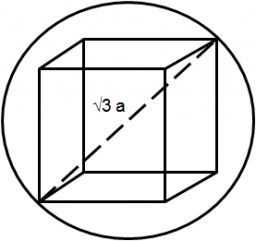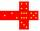# The cube

The cube has a surface of 600 cm2. What is its volume?

V =  1000 cm3

### Step-by-step explanation:Did you find an error or inaccuracy? Feel free to write us. Thank you!Tips to related online calculators
Tip: Our volume units converter will help you with the conversion of volume units.

## Related math problems and questions:

• The cubeThe cube has a surface area of 486 m ^ 2. Calculate its volume.
• Cube surfce2volumeCalculate the volume of the cube if its surface is 150 cm2.
• Area to volumeIf the surface area of a cube is 486, find its volume.
• The cubeThe cube has a surface area of 216 dm2. Calculate: a) the content of one wall, b) edge length, c) cube volume.
• Cube V2SThe volume of the cube is 27 dm cubic. Calculate the surface of the cube.
• Volume and areaWhat is the volume of a cube which has an area of 361 cm2?
• Cube edgeDetermine the edges of the cube when the surface is equal to 37.5 cm square.
• CubeThe sum of lengths of cube edges is 57 cm. What is its surface and volume?
• Cube surface and volumeThe surface of the cube is 500 cm2, how much cm3 will be its volume?
• Cuboid to cubeA cuboid with dimensions of 9 cm, 6 cm, and 4 cm has the same volume as a cube. Calculate the surface of this cube.
• Cube 6Volume of the cube is 216 cm3, calculate its surface area.
• The cubeThe surface of the cube is 150 square centimeters. Calculate: a- the content of its walls b - the length of its edges
• Edges or sidesCalculate the cube volume, if the sum of the lengths of all sides is 276 cm.
• Cube 5The content area of one cube wall is 32 square centimeters. Determine the length of its edges, its surface and volume.
• Rectangular cuboidThe rectangular cuboid has a surface area 5334 cm2, and its dimensions are in the ratio 2:4:5. Find the volume of this rectangular cuboid.
• Cuboid surfaceDetermine surface area of cuboid if its volume is 52.8 cm cubic and length of the two edges are 2 cm and 6 cm.
• Surface of cuboidFind the surface of the cuboid if its volume is 52.8 cm3 and the length of its two edges is 2 cm and 6 cm.